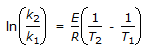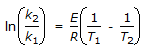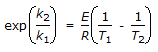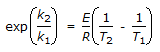# Chemical Engineering - Chemical Reaction Engineering

### Exercise :: Chemical Reaction Engineering - Section 9

11.

In an ideal mixed reactor (at steady state), the

 A. space time is equivalent to holding time for constant density systems. B. composition throughout the reactor remains same. C. exit stream has the same composition as the fluid within the reactor. D. all (a), (b) and (c).

Explanation:

No answer description available for this question. Let us discuss.

12.

The knowledge of initial concentration and rate constant is necessary to determine the half life time of a reaction of __________ order.

 A. zero B. first C. second D. none of these

Explanation:

No answer description available for this question. Let us discuss.

13.

Which of the following factors control the design of a fluid-solid reactor ?

 A. Reaction kinetics for single particle, B. Size distribution of solids beingtreated. C. Flow patterns of solids and fluid in the reactor. D. All (a), (b) and (c).

Explanation:

No answer description available for this question. Let us discuss.

14.

A Catalyst

 A. increases the equilibrium concentration of the product. B. changes the equilibrium constant of the reaction. C. shortens the time to reach the equilibrium. D. none of these.

Explanation:

No answer description available for this question. Let us discuss.

15.

The reaction rate constants at two different temperature T1 and T2 are related by

 A.B.C.D.